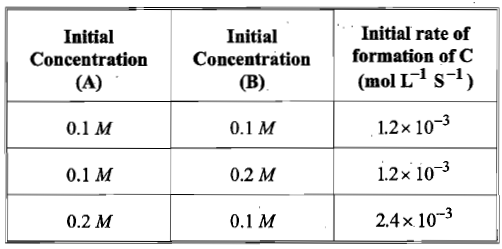# NEET Chemistry Chemical Kinetics Questions Solved

For the non-stoichiometric reaction 2A + B $\to$ C +D, the following kinetic data were obtained in three separate experiments, all at 298 K.The rate law for the formation of C is:

(a) d[C]/dt = k[ A][B]

(b) d[C]/dt = k[ A]2[B]

(c) d[C]/dt = k[ A][B]2

(d) d[C]/dt = k[ A]